Excel Pivot Tables have a lot of useful calculations under the SHOW VALUES AS option and one that can help you a lot is the Excel Formula to Calculate Percentage of Grand Total.

This option will immediately calculate the percentages for you from a table filled with numbers such as sales data, expenses, attendance, or anything that can be quantified.

Want to Show Excel Pivot Table calculated field percentage of total?

Watch it on YouTube and give it a thumbs up 👍#### Follow the step-by-step tutorial on how to show you an Excel Formula to Calculate Percentage of Grand Total and download this Excel workbook to practice along:

STEP 1: Select any cell in the data table.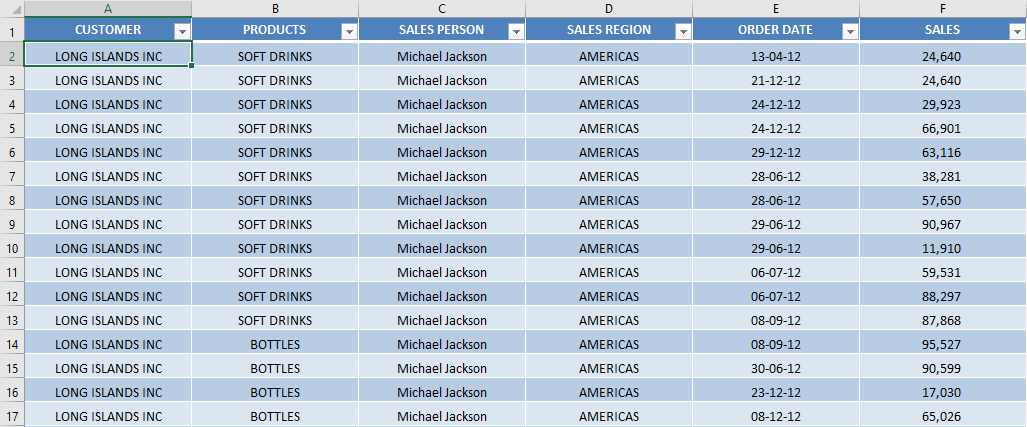STEP 2: Insert a new Pivot table by clicking on your data and going to Insert > Pivot Table.STEP 3: Insert a new Pivot In the Create PivotTable dialog box, select the table range and New Worksheet, and then click OK.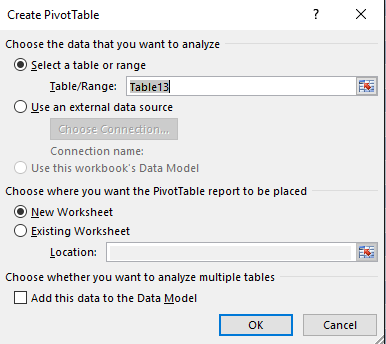STEP 4: In the ROWS section put in the Sales Month field, in the COLUMNS put in the Financial Year field and in the VALUES area you need to put in the Sales field twice, I explain why below: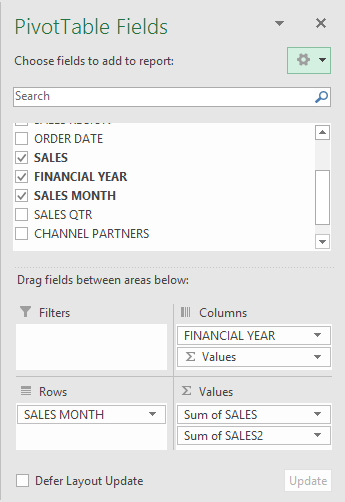The Pivot Table will look like this: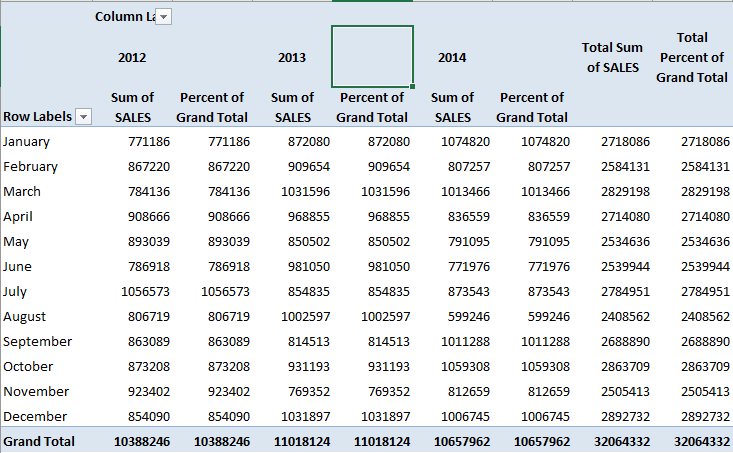STEP 5: Click the second Sales field’s (Sum of SALES2) drop down and choose Value Field SettingsSTEP 6: Select the Show Values As tab and from the drop-down choose % of Grand Total.

Also, change the Custom Name into Percent of Grand Total to make it more presentable. Click OK.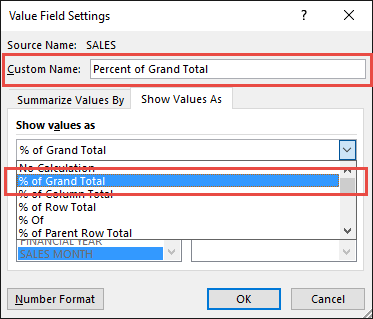An Excel formula to calculate percentage of grand total will be added!

STEP 7: Notice that the Percent of Grand Total data is in a decimal format and it is hard to read it: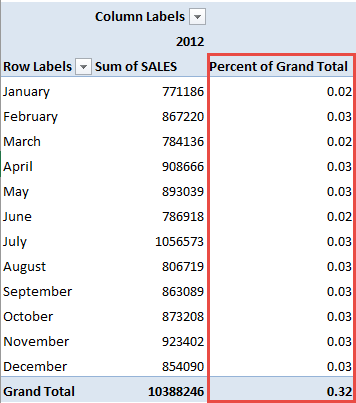To format the Percent of Grand Total column, click the second Sales field’s (Percent of Grand Total) drop down and choose Value Field Settings.

The goal here is for us to transform numbers from a decimal format (i.e. 0.23), into a percentage format that is more readable (i.e. 23%).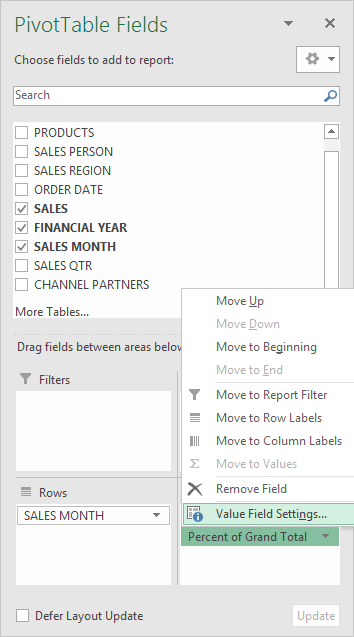STEP 8: Click the Number Format button.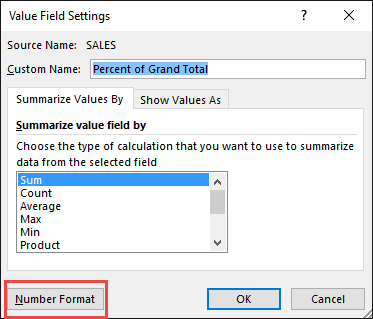STEP 9: Inside the Format Cells dialog box, make your formatting changes within here and press OK twice.

In this example, we used the Percentage category to make our Percent of Grand Total numbers become more readable.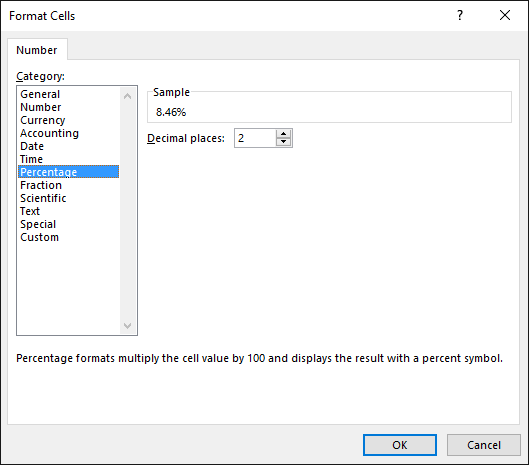You now have your Pivot Table, showing the Excel Pivot Table percentage of totalfor the sales data of years 2012, 2013, and 2014.

All of the sales numbers are now represented as a Percentage of the Grand Total of \$32,064,332.00, which you can see on the lower right corner is represented as 100% in totality: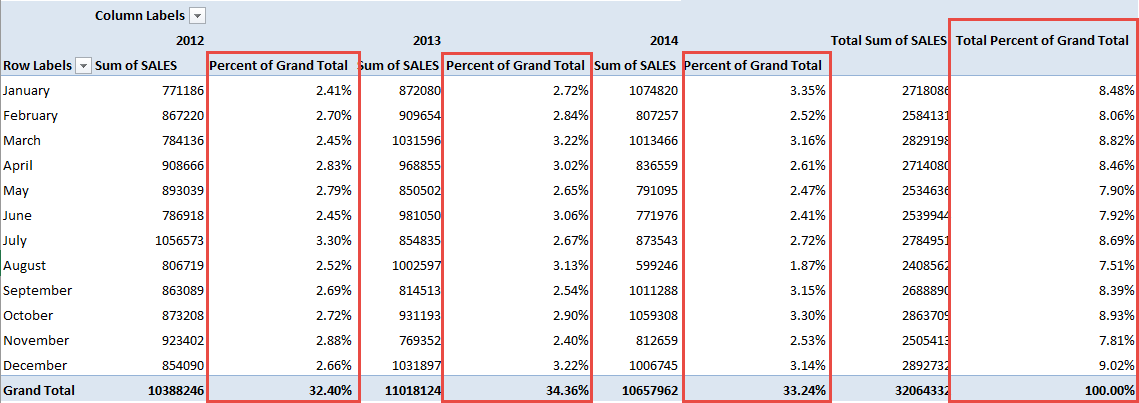This completes our tutorial on the Excel formula to calculate percentage of grand total!

You can even use this option to show the sales by category as a percentage of the grand total.